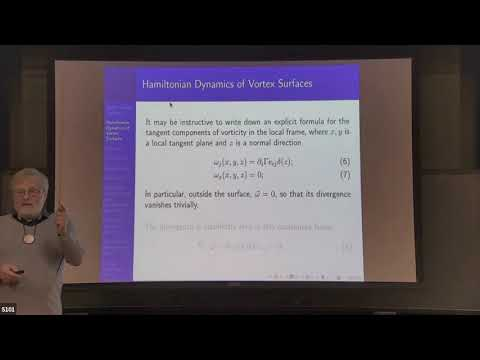### Videos

#### Stable Vortex Sheets and Irreversibility of Turbulence

##### Presenter
December 7, 2021##### Abstract
New kinds of vortex sheets with vorticity confined to the boundary layer are proposed and investigated in detail. Exact solutions of the steady Navier-Stokes equations for a planar vortex sheet in arbitrary background strain are found in terms of hypergeometric functions.These solutions are stable only under certain conditions on background strain.   The stability conditions (CVS equations) reduce to vanishing component of the local strain in the direction of the velocity gap, in addition to the usual requirement of vanishing normal velocity on each side. The normal component of the strain must be negative for stability, which is the origin of an irreversibility of Turbulence in our theory.   Anomalous energy dissipation (surface enstrophy) is conserved on CVS vortex sheets in the Navier-Stokes equation.   The CVS equations serve as boundary conditions to the Euler equations and also determine the shape of the surface up to a few parameters. These parameters are the eigenvalues of the background strain plus location and rotation matrix.   We argue that this background strain is a random gaussian symmetric traceless matrix with the calculable distribution of eigenvalues. The variance of this Gaussian tensor is related to the energy dissipation by self-consistency equations of mean-field theory.   The fluctuating ratio of eigenvalues leads to multifractal properties of the vortex surface, offering a possible explanation of the multifractal scaling laws for velocity differences in extreme turbulence.   The anomalous energy dissipation has a universal probability distribution in our theory, which is calculated numerically.